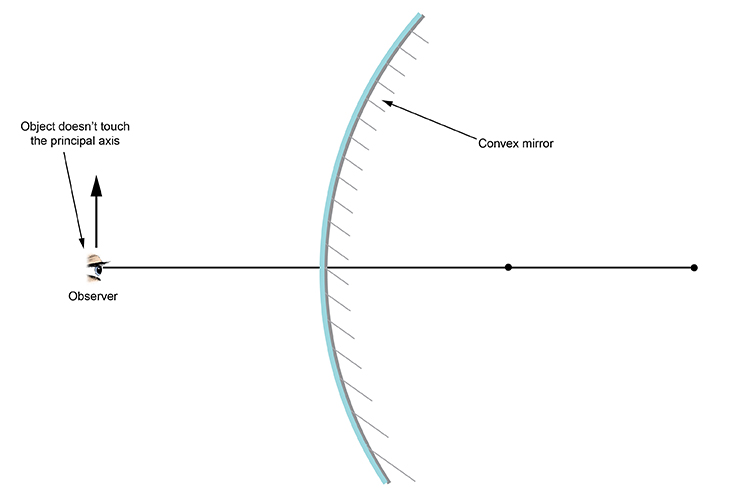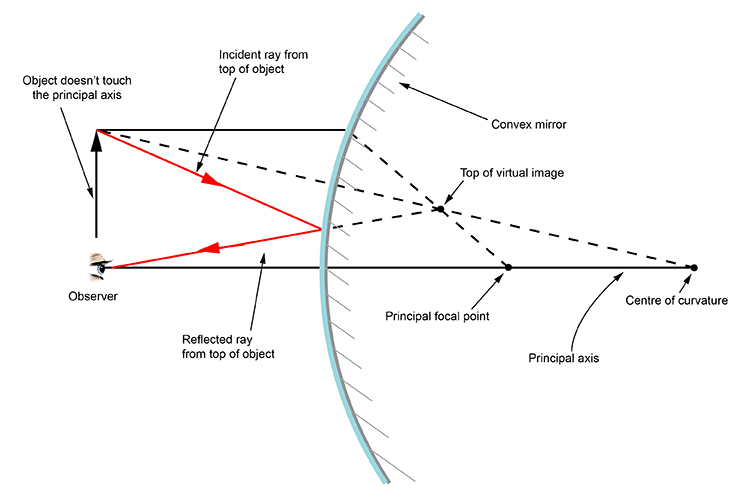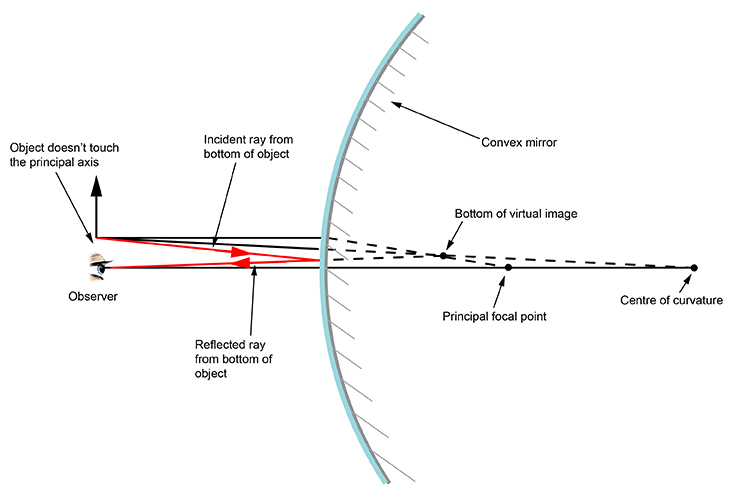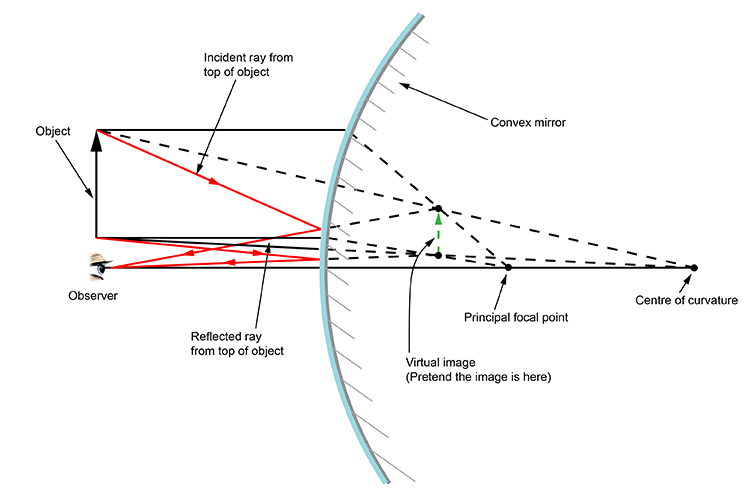# Object doesn't touch the principal axis

If the object doesn't touch the principal axis then we can still apply the two rules of reflection for a convex mirror in order that we can draw the ray diagrams.

The two rules of reflection for a convex mirror are:

From the object
1. Pass a parallel line through the principal focal point
2. Pass a ray line through the centre of the convex mirror

Here is the problemThe first stage is to apply the two rules of reflection for convex mirrors to the top of the object,

as follows:The second stage is to apply the two rules of reflection for convex mirrors to the bottom of the object,

as follows:Now if you combine stages 1 and 2 you will gain the following diagram: